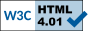Todo List
Global em_bme_3plm (int nbr_par, int max_em_iter, int max_nr_iter, double prec, gsl_matrix_int *patterns, gsl_vector *counts, gsl_vector *quad_points, gsl_vector *quad_weights, int slope_prior, double slope_mean, double slope_dev, int thresh_prior, double thresh_mean, double thresh_dev, int asymp_prior, double asymp_mean, double asymp_weight, gsl_vector *slopes, gsl_vector *thresholds, gsl_vector *asymptotes, gsl_vector *slopes_stddev, gsl_vector *thresh_stddev, gsl_vector *asymp_stddev, gsl_vector_int *ignore, int *nbr_notconverge, gsl_vector_int *notconverge, int adjust_weights)
Check for the biggest change in the EM iterations and add a stop criterion.
Global em_mple_wave (int max_em_iter, int max_nr_iter, double prec, double smooth_factor, gsl_matrix_int *patterns, gsl_vector *counts, gsl_vector *quad_points, gsl_vector *quad_weights, gsl_matrix *probs, gsl_matrix *probs_stddev, gsl_vector_int *ignore, int *nbr_notconverge, gsl_vector_int *notconverge, int adjust_weights)
Compute more accurates wavelets derivatives
Global em_mple_wave_mc (int max_em_iter, int max_nr_iter, double prec, double smooth_factor, gsl_matrix_int *patterns, gsl_vector *counts, gsl_vector *quad_points, gsl_vector *quad_weights, gsl_vector_int *items_pos, gsl_vector_int *nbr_options, gsl_matrix *probs, gsl_matrix *probs_stddev, gsl_vector_int *ignore, int *nbr_notconverge, gsl_vector_int *notconverge, int adjust_weights)
Compute more accurates wavelets derivatives
Global em_mple_wave_mc (int max_em_iter, int max_nr_iter, double prec, double smooth_factor, gsl_matrix_int *patterns, gsl_vector *counts, gsl_vector *quad_points, gsl_vector *quad_weights, gsl_vector_int *items_pos, gsl_vector_int *nbr_options, gsl_matrix *probs, gsl_matrix *probs_stddev, gsl_vector_int *ignore, int *nbr_notconverge, gsl_vector_int *notconverge, int adjust_weights)
Compute more accurates wavelets derivatives
Global frequencies (gsl_matrix_int *patterns, gsl_vector *counts, gsl_matrix *post, gsl_matrix *probs, gsl_vector *quad_sizes, gsl_matrix *quad_freqs)
Recomputing the quad_size for each item is unecessary.
page Main Page

A graded version of the PMMLE with a common logit and an "intercept" for each boundary (with zero mean)

Different models on each item

DIF

Equating

Dimensionality test

Bidimensional latent variable

Continuous itemGenerated on Sun Jan 26 2014 15:27:27 for libirt by Doxygen.